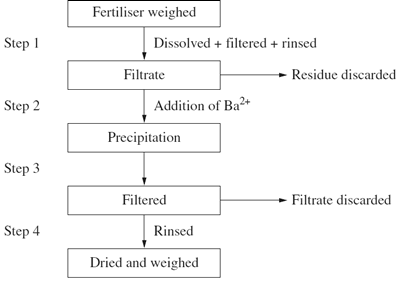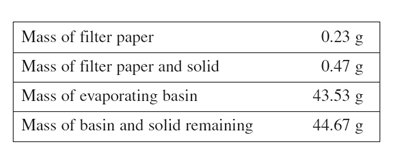Gravimetric analysis (2010 NSW HSC) 1) The flowchart shown outlines the process used to determine the amount of sulfate present in a sample of lawn fertiliser.a) What assumptions were made and how do these affect the validity of this process? Solution (b) It was found that 4.25 g had a sulfate content of 35%. What is the mass of the dried precipitate at Step 4? Include a chemical equation in your answer. Solution 2) A student collected a 250 mL sample of water from a local dam for analysis. The data collected are shown in the table.(i) The water was filtered and the filtrate evaporated to dryness. Calculate the percentage of the total dissolved solids in the dam sample. Solution (ii) It is suspected that the water in the dam has a high concentration of chloride ions. Describe a chemical test that could be carried out on the water sample to determine the presence of chloride ions. Include an equation in youranswer. Solution (iii) Name an ion other than chloride that commonly pollutes waterways, and identify its source and the effect of its presence on water quality. Solution